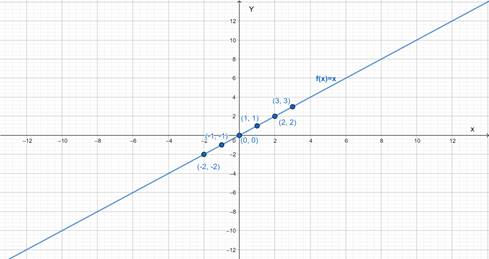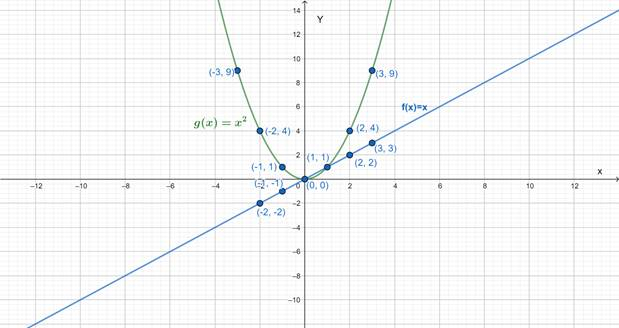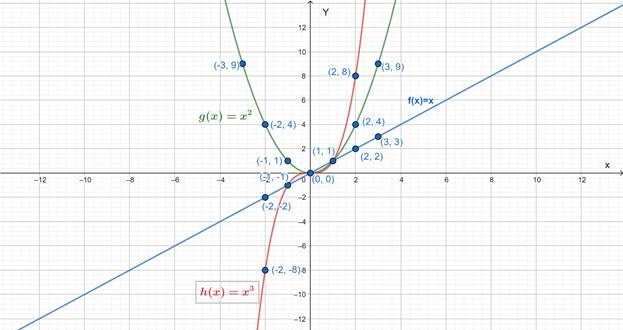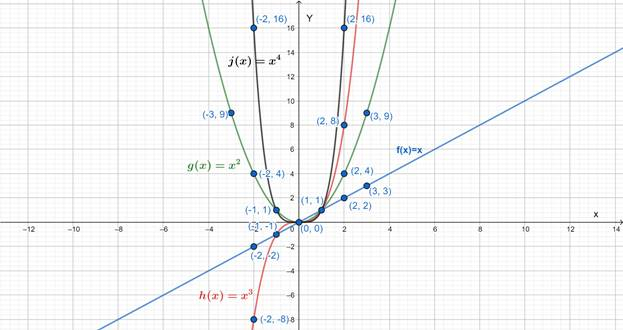# To graph : The graph of the given function.### Precalculus: Mathematics for Calcu...

6th Edition
Stewart + 5 others
Publisher: Cengage Learning
ISBN: 9780840068071### Precalculus: Mathematics for Calcu...

6th Edition
Stewart + 5 others
Publisher: Cengage Learning
ISBN: 9780840068071

#### Solutions

Chapter 2, Problem 2RCC

a.

To determine

## To graph: The graph of the given function.

Expert Solution

### Explanation of Solution

Given information: f(x)=x

Graph:

 x 0 1 2 3 −1 −2 f(x) 0 1 2 3 −1 −2Interpretation: The graph of f(x)=x is a straight line passing through origin.

b.

To determine

Expert Solution

### Explanation of Solution

Given information: g(x)=x2

Graph:

 x 0 1 2 3 −1 −2 -3 f(x) 0 1 4 9 1 4 9Interpretation: The curve in green color is the graph of g(x)=x2 .

c.

To determine

Expert Solution

### Explanation of Solution

Given information: h(x)=x3

Graph:

 x 0 1 2 −1 −2 f(x) 0 1 8 −1 −8Interpretation: The curve in red color is the graph of h(x)=x3 .

d.

To determine

Expert Solution

### Explanation of Solution

Given information: j(x)=x4

Graph:

 x 0 1 2 −1 −2 f(x) 0 1 16 1 16Interpretation: The curve in black color is the graph of j(x)=x4 .

### Have a homework question?

Subscribe to bartleby learn! Ask subject matter experts 30 homework questions each month. Plus, you’ll have access to millions of step-by-step textbook answers!